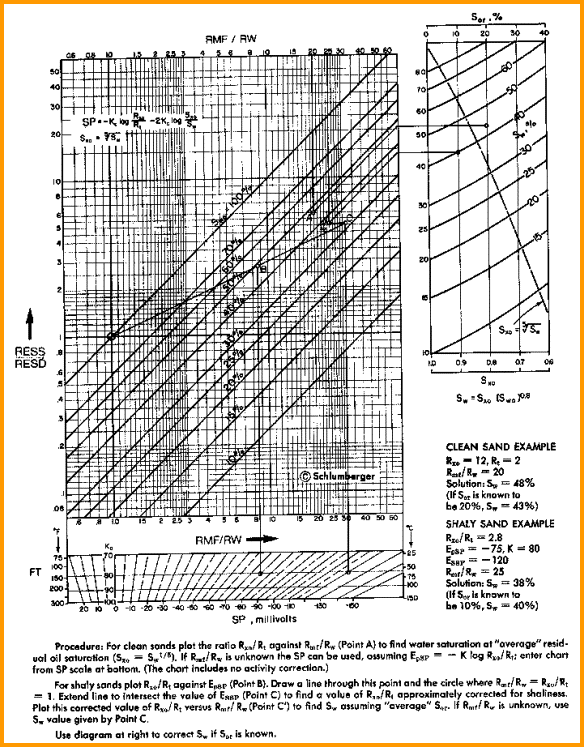Water Saturation from Ratio Method
When no porosity data is available, saturation can be obtained by comparing the shallow and deep resistivity logs. This formula is not shale corrected.

1: SWrt = SXO * ((RXO / RESD) / (RMF@FT / RW@FT)) ^ (1 / N)

Where:
N = saturation exponent (fractional)
RESD = resistivity reading on deep log (ohm-m)
RXO = resistivity reading on shallow log (ohm-m)
RMF@FT = mud filtrate resistivity (ohm-m)
RW@FT = formation water resistivity at same temperature as RMF (ohm-m)
SWrt = water saturation from ratio method (fractional)
SXO = estimated water saturation in invaded zone (fractional)

A common assumption is that Sxo = Sw^(1/5). Substituting this into equation 1 and assuming N = 2, we get:
2: SWrt = ((RXO / RESD) / (RMF@FT / RW@FT)) ^ (5/8)COMMENTS:
See below for a graphical solution to this formula. The method is a last resort to be used when no porosity log is available.

If there is a nearby water zone AND it has the same porosity as the pay zone, the Rmf/Rw ratio can be estimated from the Rxo/Rt ratio in the water zone, and used in equation 1 or 2, thus exact knowledge of Rw is not required.Graphical solution for Ratio method (with shale correction from SP)RECOMMENDED PARAMETERS:
N = 2.0
for water zone SXO = 1.00
for hydrocarbon zone with high porosity SXO = 0.60
for hydrocarbon zone with medium porosity SXO = 0.70
for hydrocarbon zone with low porosity SXO = 0.80
for heavy oil and tar sands, SXO = SW = 0.10 to 0.30

NUMERICAL EXAMPLE:
1. For Sand C:
RESD = 1.0 ohm-m
RESS = 2.0 ohm-m
RMF = 0.75 @ 25 degrees C
RMF@FT = 0.54 @ 43 degrees C
RW@FT = 0.036 @ 43 degrees C
N = 2.00
Swr = (2.0 / 1.0) / (0.54 / 0.036) ^ (1 / 2) = 0.36

Page Views ---- Since 01 Jan 2015
Copyright 2023 by Accessible Petrophysics Ltd.
CPH Logo, "CPH", "CPH Gold Member", "CPH Platinum Member", "Crain's Rules", "Meta/Log", "Computer-Ready-Math", "Petro/Fusion Scripts" are Trademarks of the Author